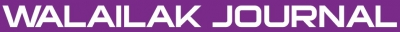### A New Mathematical Model for Nonlinear Wave in a Hyperelastic Rod and Its Analytic Approximate Solution

Sunil KUMAR

#### Abstract

This paper proposes a fractional model for nonlinear waves in hyperelastic rods, which describes far-field, finite length, finite amplitude radial deformation waves in cylindrical compressible hyperelastic rods. In this model, fractional derivatives are described in the Caputo sense. The error analysis shows that our approximate solution converges very rapidly to the exact solution and the numerical solution is compared with the known analytical solution which is nearly identical with the exact solution. The method introduces a promising tool for solving time the fractional hyperelastic rod equation.

doi:10.14456/WJST.2014.71

#### Keywords

Hyperelastic rod, fractional derivative, analytic approximate solution, homotopy perturbation method

PDF

#### References

HH Dai. Exact travelling-wave solutions of an integrable equation arising in Hyperelastic rods. Wave Motion 1998; 28, 367-81.

HH Dai and Y Huo. Solitary shock waves and other travelling waves in a general compressible hyperelastic rod. Proc. R. Soc. Lond. A 2000; 456, 331-63.

R Camassa and DD Holm. An integrable shallow wave equation with peaked solitons. Phys. Rev. Lett. 1993; 71, 1661-4.

RS Johnson and C Holm. Korteweg-de Vries and related models for water waves. J. Fluid Mech. 2002; 455, 63-82.

TB Benjamin, JL Bona and JJ Mahony. Model equations for long waves in nonlinear dispersive systems. Philos. Trans. R. Soc. Lond. A 1972; 272, 47-78.

TB Benjamin. The stability of solitary waves. Proc. Roy. Soc. Lond. A 1972; 328, 153-83.

A Constantin and WA Strauss. Stability of a class of solitary waves in compressible elastic rods. Phys. Lett. A 2000; 270, 140-8.

M Bendahamane, GM Coclite and KH Karlsen. H1-perturbations of smooth solution for a weakly Dissipative Hyperelastic-Rod wave equation. Mediterr. J. Math. 2006; 3, 419-32.

J Li and Y Zang. Exact travelling wave solutions in a nonlinear elastic rod equation. Appl. Math. Comput. 2008; 2002, 504-10.

L Zhengrong and Z Bengong. New expressions of periodic waves and a novel phenomenon in a compressible Hyperelastic rod. J. Partial. Diff. Eqs. 2007; 20, 80-96.

H Holden and X Raynaud. Global conservative solutions of the generalized Hyperelastic rod equation. J. Differ. Equat. 2007; 233, 448-84.

GM Coclite, H Holden and KH Karlsen. Global weak solutions to a generalized Hyperelastic rod wave equation. SIAM J. Math Anal. 2005; 37, 1044-69.

L Jin, Y Liu and Y Zhou. Blow up of solutions to a periodic nonlinear dispersive rod equation. Documenta Math. 2010; 15, 267-83.

E Wahlen. On the blow up of solutions to a nonlinear dispersive rod equation. J. Math. Anal. Appl. 2006; 323, 1318-24.

BJ West, M Bolognab and P Grigolini. Physics of Fractal Operators. Springer, New York, 2003.

I Podlubny. Fractional Differential Equations Calculus. Academic Press, New York, 1999.

KB Oldham and J Spanier. The Fractional Calculus. Academic Press, New York, 1974.

KS Miller and B Ross. An Introduction to the Fractional Calculus and Fractional Differential Equations. John Willey and Sons, New York, 2003.

AA Kilbas, HM Srivastava and JJ Trujillo. Theory and Applications of Fractional Differential Equations. Elsevier, Amsterdam, 2006.

K Diethelm and NJ Ford. Analysis of fractional differential equations. J. Math. Anal. Appl. 2002; 265, 229-48.

K Diethelm. An algorithms for the numerical solutions of differential equations of fractional order. Elec. Trans. Numer. 1997; 5, 1-6.

SG Samko, AA Kilbas and OI Marichev. Fractional Integrals and Derivatives: Theory and Applications. Yverdon. Gordon and Breach. 1993.

JH He. Homotopy perturbation technique. Comput. Meth. Appl. Mech. Eng. 1999; 178, 257-62.

JH He. A coupling method of a homotopy technique and a perturbation technique for non-linear problems. Int. J. Nonlinear Mech. 2000; 35, 37-43.

JH He. Some asymptotic methods for strongly nonlinear equations. Int. J. Mod. Phys. B 2006; 20, 1141-99.

JH He. A new perturbation technique which is also valid for large parameters. J. Sound Vibration 2000; 229, 1257-63.

A Yildirim and H Kocak. Homotopy perturbation method for solving the space time fractional Advection dispersion equation. Adv. Water. Resour. 2009; 32, 1711-6.

A Yildirim and Y Gulkanat. Analytical approach to fractional Zakharov-kuznetsov equations by He’s homotopy perturbation method. Comm. Theor. Phys. 2010; 53, 1005-10.

S Kumar, Y Khan and A Yildirim. A mathematical modelling arising in the chemical systems and its approximate numerical solution. Asia Pacific J. Chem. Eng. 2012; 7, 835-40.

S Kumar, H Kocak and A Yildirim. A fractional model of gas dynamics equation by using Laplace transform. Z. Naturforsch. 2012; 67, 389-96.

S Kumar and OP Singh. Inversion of Abel integral equation by homotopy perturbation method. Z. Naturforsch. 2010; 65, 677-82.

S Kumar, M Tripathi and OP Singh. A fractional model of Harry Dym equation and its approximate solution. Ain Shams Eng. J. 2013; 4, 111-5.

S Momani and Z Odibat. Homotopy perturbation method for nonlinear partial differential equations of fractional order. Phys. Lett. A 2007; 365, 345-50.

A Yildirim, H Askari, MK Yazdi and Y Khan. A relationship between three analytical approaches to nonlinear problems. Appl. Math. Lett. 2012; 25, 1729-33.

D Younesian, H Askari, Z Saadatnia and MK Yazdi. Analytical approximate solutions for the generalized nonlinear oscillator. Applicable Anal. 2012; 91, 965-77.

A Yildirim, H Askari, Z Saadatnia, MK Yazdi and Y Khan. Analysis of nonlinear oscillations of a punctual charge in the electric field of a charged ring via a Hamiltonian approach and the energy balance method. Comput. Math. Appl. 2011; 62, 486-90.

D Younesian, H Askari, Z Saadatnia and A Yildirim. Analytical solution for nonlinear wave propagation in shallow media using the variational iteration method. Waves Random Complex Media 2012; 22, 133-42.

D Younesian, H Askari, Z Saadatnia and A Yildirim. Periodic solutions for the generalized nonlinear oscillators containing fraction order elastic force. Int. J. Nonlinear Sci. Numer. Simulat. 2011; 11, 1027-32.

Y Luchko and R Gorneflo. The Initial Value Problem for Some Fractional Differential Equations with the Caputo Derivatives. Preprint series A08-98, Fachbreich Mathematick und Informatik, Freic Universitat Berlin, 1998.

### Refbacks

• There are currently no refbacks.Online ISSN: 2228-835X

http://wjst.wu.ac.th

Last updated: 20 June 2019